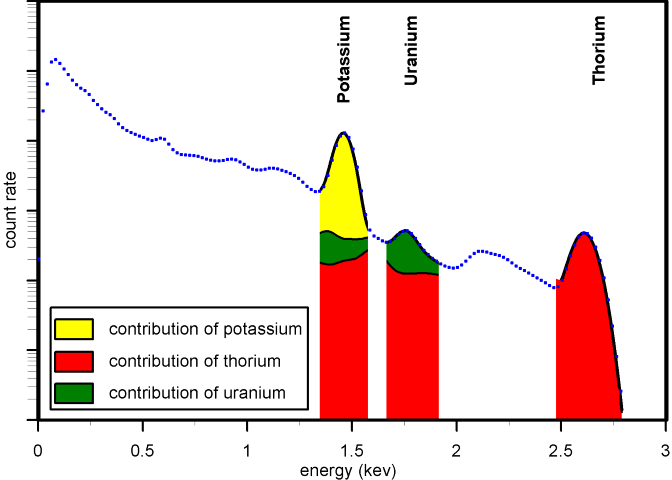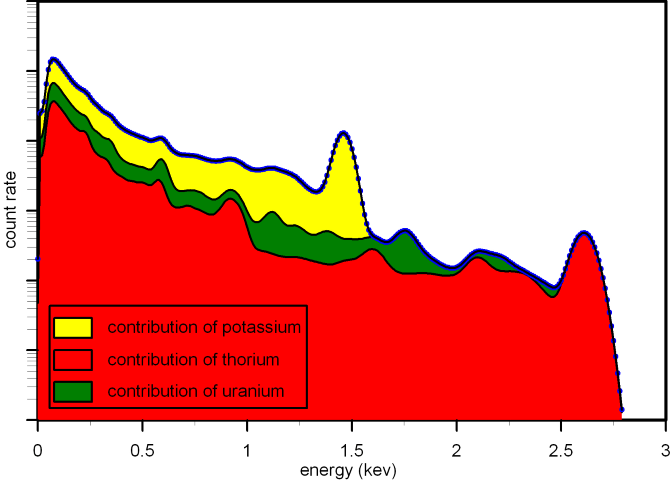# 13. What is the "theoretical" count rate in Gamman?

Count rate can be defined as the number of counts per second collected by a gamma spectrometer. Problem with this "raw" count rate is that it is not well defined. The hardware will have a lower level and does not count any gamma particles below this level. When temperature changes, the whole spectrum will shift, but the lower level will not be affected. As a result, the "raw" count rate is temperature dependent. The high voltage applied to the spectrometer has a similar effect. This effect is shown in more detail here.

Gamman offers the possibility to calculate a "theoretical" count rate, which is not affected by the factors mentioned above. To get to a total count rate, basically you have to sum the activities (in Bq/kg) for each nuclide in the source multiplied with the content of the standard spectra for these nuclides. The standard standard spectra are determined during the calibration process and are defined as the response curves for 1 Bq/kg during a measurement duration of 1 second. Normally, the spectrum content is determined over the analysis interval only (typically from 300 keV to 2800 keV). Alternatively, one could use the full energy interval. In the latter form, the count rate found will be closer to the "raw" count rate one would have measured.

As an example, suppose that we measure 100 Bq/kg of 40K, 10 Bq/kg 232Th and 20 Bq/kg 238U. The standard spectra contain 1, 10 and 10 counts per second over the analysis interval. The theoretical count rate in this situation would be 100 * 1 + 10 * 10 + 20 * 10 = 400 counts per second.

The "theoretical" count rates can also be determined by using small windows around the photo peaks (see the figures below). Using the full spectrum gives higher count rates (and better statistics) as compared to this approach.JavaScript errors detected